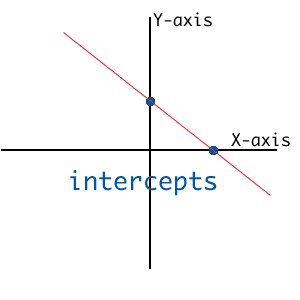my name is nay 8th grade student how do i figure out an intercep and does it always have to have (0, ) or ( ,0)? Hi Nay, When you graph a line or a curve, the intercepts are the points where the line or curve touches the X or Y axes. Thus, in the diagram below, the intercepts are the blue dots. The X-intercept is the point on the X-axis and the Y-intercept is the point on the Y-axis.Every point on the X-axis is of the form (p,0) for some number p and every point on the Y-axis has the form (0,q) for some number q. Thus the answer to your question is yes, intercepts have to be (,0) or (0,) Thus, if you have the equation of a line, say y = 5x - 3 then you know the Y-intercept is (0,q) for some number q, and thus x=0. Hence if you let x=0 in the equation you get y = 5(0) - 3 = -3 Thus the Y-intercept is (0,-3). Likewise the X-intercept is (p,0) for some p, and hence if you put y=0 in the equation you get 0 = 5x -3 and thus x = 3/5 Thus the X-intercept is (3/5,0) Cheers, Penny Go to Math Central